## How to Calculate and Solve for Mass, Volume and Linear Momentum | The Calculator Encyclopedia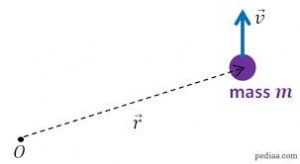The image above represents a linear momentum.

To compute for the linear momentum, two essential parameters are needed and these parameters are mass (m) and velocity (v).

The formula for calculating linear momentum:

p = mv

Where;
p = Momentum
m = Mass
v = Velocity

Let’s solve an example;
Find the linear momentum of a mass of 44 and a velocity of 38.

This implies that;
m = Mass = 44
v = Velocity = 38

p = mv
p = 44 x 38
p = 1672

Therefore, the linear momentum is 1672 Kgm/s.

Calculating the Mass when Linear Momentum and Velocity is Given.

m = p / v

Where;
m = Mass
p = Momentum
v = Velocity

Let’s solve an example;
Find the mass with a linear momentum of 320 and a velocity of 80.

This implies that;
p = Momentum = 320
v = Velocity = 80

m = p / v
m = 320 / 80
m = 4

Therefore, the mass is 4 kg.

## How to Calculate and Solve for the Quantity of Charge, Electrochemical Equivalence of a Substance and Mass of an Element in Electrolysis | Nickzom Calculator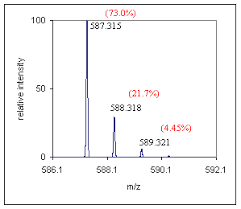The image above represents the mass of an element.

To compute for the mass of an element, two essential parameters are needed and these parameters are Electrochemical Equivalence of the Substance (Z) and quantity of charge (Q).

The formula for calculating mass of an element:

M = ZQ

Where;
M = Mass of the element
Z = Electrochemical Equivalence of the Substance
Q = Quantity of Charge

Let’s solve an example;
Find the mass of an element when the Quantity of charge is 28 and Electrochemical Equivalence of the Substance is 32.

This implies that;
Z = Electrochemical Equivalence of the Substance = 32
Q = Quantity of Charge = 28

M = ZQ
M = 32 x 28
M = 896

Therefore, the mass of an element is 896 kg.

Calculating the Electrochemical Equivalence of the Substance when the Mass of an Element and Quantity of Charge is Given.

Z = M / Q

Where;
Z = Electrochemical Equivalence of the Substance
M = Mass of the element
Q = Quantity of Charge

Let’s solve an example;
Find the Electrochemical Equivalence of the Substance when the Quantity of charge is 12 and  mass of an element  is 120.

This implies that;
M = Mass of the element = 120
Q = Quantity of Charge = 12

Z = M / Q
Z = 120 / 12
Z = 10

Therefore, the Electrochemical Equivalence of the Substance is 10.

## How to Calculate and Solve for the Current, Time and Quantity of Charge of an Electrolysis | The Calculator Encyclopedia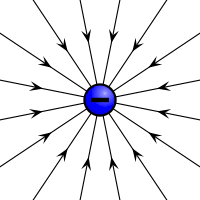The image above represents the quantity of charge.

To compute for the quantity of charge, two essential parameters are needed and these parameters are current (I) and time (T).

The formula for calculating the quantity of charge:

Q = It

Where;
Q = Quantity of charge
I = Current
T = Time

Let’s solve an example;
Find the quantity of charge with a current of 24 and time of 12.

This implies that;
I = Current = 24
T = Time = 12

Q = It
Q = 24 x 12
Q = 288

Therefore, the quantity of charge is 288 Coulombs (C).

Calculating the Current (I) using the Quantity of Charge and Time.

I = Q / t

Where;
I = Current
Q = Quantity of charge
T = Time

Let’s solve an example;
Given that the quantity of charge is 240 with a time of 14. Find the Current?

This implies that;
Q = Quantity of charge = 240
T = Time = 14

I = Q / t
I = 240 / 14
I = 17.14

Therefore, the current is 17.14 ampere.

## How to Calculate and Solve for Van’t Hoff Factor, Ebullioscopic Constant, Molality and Boiling Point Elevation | The Calculator Encyclopedia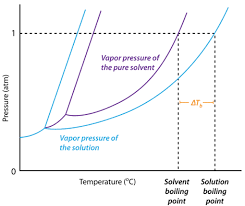The image above represents boiling point elevation.

To compute for the boiling point elevation, three parameters are needed and these parameters are Van’t Hoff’s Factor (i), ebullioscopic constant (Kb) and Molality.

The formula for calculating boiling point elevation:

δTb = iKb x Molality

Where;
δTb = boiling point elevation
i = Van’t Hoff’s Factor
Kb = ebullioscopic constant
Molality

Let’s solve an example;
Find the boiling point elevation when the Van’t Hoff’s Factor is 42, ebullioscopic constant is 60 and molality of 180.

This implies that;
i = Van’t Hoff’s Factor = 42
Kb = ebullioscopic constant = 60
Molality = 180

δTb = iKb x Molality
δTb = (42)(60) x 180
δTb = (2520) x 180
δTb = 453600

Therefore, the boiling point elevation is 453600 °C m-1.

Calculating the Molality using the Boiling Point Elevation, Van’t Hoff’s Factor and Ebullioscopic Constant.

Molality = δTb / iKb

Where;
Molality
δTb = boiling point elevation
i = Van’t Hoff’s Factor
Kb = ebullioscopic constant

Let’s solve an example;
Find the molality with a boiling point elevation of 120 and a van’t hoff’s factor of 32 with a ebullioscopic constant of 12.

This implies that;
δTb = boiling point elevation = 120
i = Van’t Hoff’s Factor = 32
Kb = ebullioscopic constant = 12

Molality = δTb / iKb
Molality = 120 / 384
Molality = 0.3125

Therefore, the molality is 0.3125.

## How to Calculate and Solve for Van’t Hoff’s Factor, Cryoscopic Constant, Molality and Freezing Point Depression | Nickzom Calculator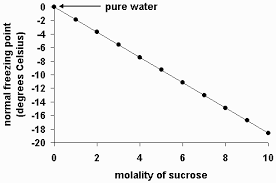The image above represents the freezing point depression.

To compute for the freezing point depression, three essential parameters are needed and these parameters are Van’t Hoff’s Factor (i), cryoscopic constant (Kf) and molality.

The formula for calculating freezing point depression:

δTf = iKf x Molality

Where;
δTf = Freezing point depression
i = Van’t Hoff’s Factor
Kf = cryoscopic constant
Molality

Let’s solve an example;
Find the freezing point depression when the van’t hoff’s factor is 12, cryoscopic constant is 21 with a molality of 16.

δTf = iKf x Molality
δTf = (12 x 21) x 16
δTf = 252 x 16
δTf = 4032

Therefore, the freezing point depression is 4032 °C m-1.

## How to Calculate and Solve for Mass, Volume and Density | The Calculator Encyclopedia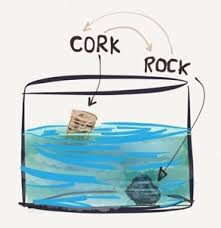The image above represents density.

To compute for the density, two essential parameters are needed and these parameters are mass (m) and volume (v).

The formula for calculating density:

Density = mass / volume

Let’s solve an example;
Given that the volume is 20 m³ with a mass of 240 kg. Find the density?

This implies that;
Volume = 20
Mass = 240

Density = mass / volume
Density = 240 / 20
Density = 12

Therefore, the density is 12 Kg/m³.

Calculating the Mass when the Density and Volume is Given.

Mass = Volume x Density

Let’s solve an example;
With a density of 90 kg/m³ and a volume of 15 m³, Find the mass?

This implies that;
Density = 90
Volume = 15

Mass = Volume x Density
Mass = 15 x 90
Mass = 1350

Therefore, the mass is 1350 kg.

## How to Calculate and Solve for Percentage Yield, Actual Yield and Theoretical Yield in Chemistry | The Calculator Encyclopedia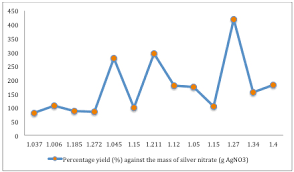The image above represents percentage yield.

To compute the percentage yield, two essential parameters are needed and these parameters are actual yield and theoretical yield.

The formula for calculating percentage yield:

%Yield = (Actual YieldTheoretical Yield) x 100

Let’s solve an example;
Find the percentage yield when the actual yield is 12 with a theoretical yield of 26.

This implies that;
Actual yield = 12
Theoretical yield = 26

%Yield = (Actual YieldTheoretical Yield) x 100
%Yield = (1226) x 100
%Yield = 0.46 x 100
%Yield = 46.15

Therefore, the percentage yield is 46.15.

Calculating the Actual Yield when the Percentage Yield and the Theoretical Yield is Given.

Actual yield = %yield x theoretical yield / 100

Let’s solve an example;
Find the actual yield when the %yield is 52 with a theoretical yield of 10.

This implies that;
%yield = 52
Theoretical yield = 10

Actual yield = %yield x theoretical yield / 100
Actual yield = 52 x 10 / 100
Actual yield = 520 / 100
Actual yield = 5.2

Therefore, the actual yield is 5.2.

## How to Calculate and Solve for the Molar Concentration, Molar Mass and Mass Concentration in Chemistry | The Calculator Encyclopedia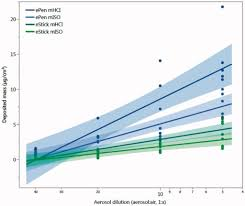The image above represents the mass concentration.

To compute for mass concentration, two essential parameters are needed and these are molar concentration (c) and molar mass (M).

ρ = c x M

Where;
ρ = mass concentration
c = molar concentration
M = molar mass

Let’s solve an example;
Find the mass concentration when the molar concentration is 24 and molar mass is 15.

This implies that;
c = molar concentration = 24
M = molar mass = 15

ρ = c x M
ρ = 24 x 15
ρ = 360

Therefore, the mass concentration is 360 Kg/dm³.

Calculating the Molar Concentration when the Mass concentration and Molar Mass.

c = ρ / M

Where;
c = molar concentration
ρ = mass concentration
M = molar mass

Let’s solve an example;
Find the molar concentration when the mass concentration is 120 with a molar mass of 40.

This implies that;
ρ = mass concentration = 120
M = molar mass = 40

c = ρ / M
c = 120 / 40
c = 3

Therefore, the molar concentration is 3 mol/L.

## How to Calculate and Solve for the Mass, Volume and Mass Concentration in Chemistry | The Calculator Encyclopedia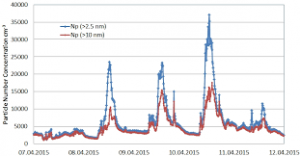The image above represents the mass concentration.

To compute the mass concentration, two essential parameters are needed and these parameters are mass (m) and volume (V).

The formula for calculating mass concentration:

ρ = mV

Where;
ρ = Mass concentration
m = Mass
V = Volume

Let’s solve an example;
Find the mass concentration when the mass is 8 kg with a volume of 24 dm³.

This implies that;
m = Mass = 8
V = Volume = 24

ρ = mV
ρ = 824
ρ = 0.33

Therefore, the mass concentration is 0.33 Kg/dm³.

Calculating the Mass when the Mass Concentration and the Volume is Given.

m = Vρ

Where;
m = Mass
ρ = Mass concentration
V = Volume

Let’s solve an example;
Find the mass when the mass concentration is 12 kg/dm³ with a volume of 7 dm³.

This implies that;
ρ = Mass concentration = 12
V = Volume = 7

m = Vρ
m = 12 x 7
m = 84

Therefore, the mass concentration is 84 kg.

## How to Calculate and Solve for the Number of Moles, Volume and Molar Concentration in Chemistry | Nickzom CalculatorThe image above represents the molar concentration.

To compute the molar concentration of a substance, two essential parameters are needed and these parameters are number of moles (n) and volume (V).

The formula for calculating the molar concentration:

c = nV

Where;
c = Molar Concentration
n = Number of moles
V = Volume

Let’s solve an example;
Find the molar concentration when the number of moles is 32 with a volume of 120.

This implies that;
n = Number of moles = 32
V = Volume = 120

c = nV
c = 32120
c = 0.266

Therefore, the molar concentration is 0.266 mol/L.

Calculating the Number of Moles when Molar Concentration and Volume is Given.

n = Vc

Where;
n = Number of moles
c = Molar Concentration
V = Volume

Let’s solve an example;
Find the number of moles when the molar concentration is 40 with a volume of 31.

This implies that;
c = molar concentration = 40
V = Volume = 31

n = Vc
n = 31 x 40
n = 1240

Therefore, the Number of Moles is 1240 mol.